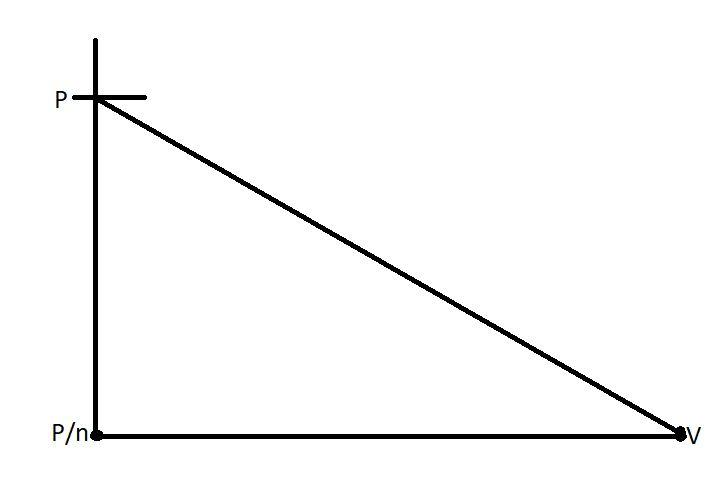QuestionAnswers

# One mole of an ideal gas at temperature T was cooled isochorically till the gas pressure fell from P to $\dfrac{P}{n}$​. Then, by an isobaric process, the gas was restored to the initial temperature. The net amount of heat absorbed by the gas in the process is\begin{align} & \text{A}\text{. nRT} \\ & \text{B}\text{. }\dfrac{RT}{n} \\ & \text{C}\text{. RT}\left( \text{1-}\dfrac{1}{n} \right) \\ & \text{D}\text{. RT(n-1)} \\ \end{align}

Hint: First draw the required graph. Now we see that the pressure is falling from pressure P to P/n, and when pressure is decreasing volume is also decreasing. Now take the formula for ideal gas. Equate those two to find how two gases are related to each other. Then find the value volume of the second gas in terms of the first one and now as per the given conditions apply it in the formula net heat.

Formula used:
PV = nRT, here P is the pressure, V is the volume, n is the number of moles, R is the universal gas constant, and T is the temperature of the gas.In the above graph we see that, the pressure is falling from p to $\dfrac{P}{n}$, and as the pressure falls, the volume also decreases to ‘V’.
We know that,
${{P}_{1}}{{V}_{1}}=nR{{T}_{1}}$ and ${{P}_{2}}{{V}_{2}}=nR{{T}_{2}}$,
We also know that this ${{T}_{1}}$and${{T}_{2}}$, both are the same.
So we can write,
${{P}_{1}}{{V}_{1}}={{P}_{2}}{{V}_{2}}$.
Now if we consider${{P}_{1}}{{V}_{1}}=PV$, then
$PV=\dfrac{P}{n}{{V}_{2}}$, as ${{P}_{2}}=\dfrac{P}{n}$ (given).
${{V}_{2}}=nV$.
Now we have to find the net heat,
We know that net heat is= nRT.
Now as off in the first condition:
$nRT=P\Delta V=\dfrac{P}{n}\left( nV-V \right)$,
$\dfrac{P}{n}V\left( n-1 \right)$, now if we replace the PV with nRT, as PV=nRT.
Then we get the result as,
$RT\left( n-1 \right)$,

Thus option D is the correct option.

Note:
An isochoric process is a thermodynamic process in which the volume of the gas remains constant in the system, whereas in the case of an isobaric process the pressure of the system always remains constant.